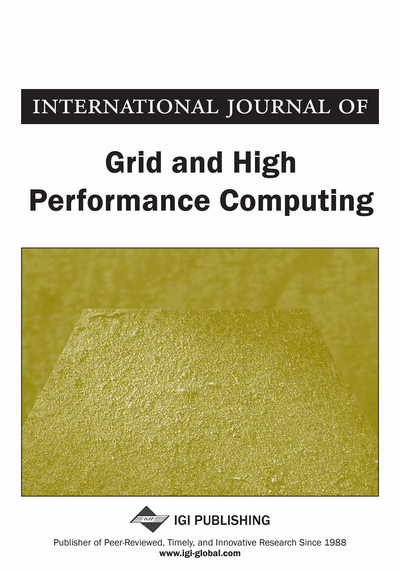# GPU Implementation of Image Convolution Using Sparse Model with Efficient Storage Format

Saira Banu Jamal Mohammed (VIT University, Vellore, India), M. Rajasekhara Babu (School of Computing Science and Engineering, VIT University, Vellore, India) and Sumithra Sriram (VIT University, Vellore, India)
DOI: 10.4018/IJGHPC.2018010104

## Abstract

With the growth of data parallel computing, role of GPU computing in non-graphic applications such as image processing becomes a focus in research fields. Convolution is an integral operation in filtering, smoothing and edge detection. In this article, the process of convolution is realized as a sparse linear system and is solved using Sparse Matrix Vector Multiplication (SpMV). The Compressed Sparse Row (CSR) format of SPMV shows better CPU performance compared to normal convolution. To overcome the stalling of threads for short rows in the GPU implementation of CSR SpMV, a more efficient model is proposed, which uses the Adaptive-Compressed Row Storage (A-CSR) format to implement the same. Using CSR in the convolution process achieves a 1.45x and a 1.159x increase in speed compared to the normal convolution of image smoothing and edge detection operations, respectively. An average speedup of 2.05x is achieved for image smoothing technique and 1.58x for edge detection technique in GPU platform usig adaptive CSR format.
Article Preview

## Introduction

Image processing involves the subjection of the source image to various operations, depending on the need of the application, to get a desired target image. The process of convolution is one of the most integral operations that are used for modifying the pixel values of the images. Various operations such as smoothing, edge-detection, sharpening, etc. can be achieved using the process of convolution. A multitude of kernels has been proposed over the years to better achieve the output image. Recently, sparse matrices have been found to play a vital role in image processing (Wang, Yan, Pan, & Xiang, 2011). Sparse matrices are matrices that are majorly populated with zeroes. The image processing operations are represented in the form of SpMV, where the sparse matrix kernel depends on the operation to be performed, and the vector is the input image represented as a one-dimensional vector. This can be easily extended to implement complex operations that involve the use of more than one of the basic processes. High efficiency gains can be obtained when all the constituent processes can be represented as a sparse matrix, and the entire image manipulating operation can be implemented as a series of SpMV operations. These complex processes however can involve multiple SpMV operations of very large matrices, depending on the size of the image and the kernel. Due to the large size of the sparse matrix kernel involved, various sparse matrix storage formats such as the Coordinate (COO) format, the Compressed Row Storage (CSR) format, the Quadtree CSR (QCSR) format, etc., can be used to further increase the space and time complexity. The CSR format has been found to be the most efficient in terms of space and time complexity of the SpMV operation. With the demand for the need for greater efficiency when using large datasets, high performance computing has become a booming area of research. Much of the concentration to achieve this is on multi-threading and graphics processing unit (GPU). An improvised version of the CSR format, the Adaptive CSR format has been recently proposed for the GPU platform which gives a considerable speedup over the implementation of the normal CSR format.

In this paper, we propose a model for the implementation of convolution for images which involves the use of SpMV using the adaptive CSR format. Results are shown for various image processing applications such as edge detection and smoothing, and it is clear that the proposed model shows considerable time gain.

## Complete Article List

Search this Journal:
Reset
Open Access Articles: Forthcoming
Volume 12: 4 Issues (2020): Forthcoming, Available for Pre-Order
Volume 11: 4 Issues (2019): 3 Released, 1 Forthcoming
Volume 10: 4 Issues (2018)
Volume 9: 4 Issues (2017)
Volume 8: 4 Issues (2016)
Volume 7: 4 Issues (2015)
Volume 6: 4 Issues (2014)
Volume 5: 4 Issues (2013)
Volume 4: 4 Issues (2012)
Volume 3: 4 Issues (2011)
Volume 2: 4 Issues (2010)
Volume 1: 4 Issues (2009)
View Complete Journal Contents Listing